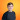2022-04-07

# Advent of Code, and the Amb OperatorThere is nothing quite like friendly competition to refuel your passions. Our team at Aha! recently sponsored 2021's Advent of Code, an annual event posing a series of programming puzzles that can be solved in any programming language you choose. We had a lot of fun going through the challenges together and comparing notes about how we solved the different problems. Our own Justin Paulson made it a few days using only Google Sheets to solve the problems! I ended up using a little-known library called `amb` for day 8's Seven Segment Search. The core of my solution was this:

``````  Ambiguous.solve do |amb|
s2 = inputs.detect {|i| i.length == 2}
s3 = inputs.detect {|i| i.length == 3}
s4 = inputs.detect {|i| i.length == 4}

a = (s3 - s2).first
b = amb.choose(*(s4 - s2))
c = amb.choose(*s2)
d = amb.choose(*%w[a b c d e f g])
e = amb.choose(*%w[a b c d e f g])
f = amb.choose(*s2)
g = amb.choose(*%w[a b c d e f g])

amb.assert [a, b, c, d, e, f, g].uniq.size == 7

# assert inputs include all possible values
amb.assert inputs.include?([a, b, c, e, f, g].sort) # 0
amb.assert inputs.include?([c, f].sort) # 1
amb.assert inputs.include?([a, c, d, e, g].sort) # 2
amb.assert inputs.include?([a, c, d, f, g].sort) # 3
amb.assert inputs.include?([b, c, d, f].sort) # 4
amb.assert inputs.include?([a, b, d, f, g].sort) # 5
amb.assert inputs.include?([a, b, d, e, f, g].sort) # 6
amb.assert inputs.include?([a, c, f].sort) # 7
amb.assert inputs.include?([a, b, c, d, e, f, g].sort) # 8
amb.assert inputs.include?([a, b, c, d, f, g].sort) # 9

# wire to display segment:
# "wire(key) b lights up segment(value) c"
wire_key = {
a => 'a',
b => 'b',
c => 'c',
d => 'd',
e => 'e',
f => 'f',
g => 'g'
}
end
``````

Amb is a neat library which provides ambiguous matching. It comes with two methods: `choose` and `assert`. `choose` gives you a value (not a proxy, but one of the real objects you pass into the `choose` operator) that you can use in `assert` clauses later on. `assert` works as you think it would. Except instead of throwing an error like `assert` would do in a test, it backtracks program execution to one of the choices, and picks a different choice.

This makes the Amb library incredibly useful for constraint-solving problems where it is easier to declare what a correct solution would look like, instead of writing out every step necessary to get there.

Unfortunately, this solution has a few drawbacks:

1. In code like this, it is not obvious that the lines between `choose` and the last `assert` might be executed many different times. If you tried using this in a production Rails application, you could imagine it being easy to insert many records into a database or have some other side effect a number of different times.
2. Continuations are deprecated in Ruby. They are only present in YARV and not in other Ruby interpreters.
3. Continuations use the language's stack and implementation details in order to manage the state of your program data. Depending on your programming philosophy, this could present big problems.

How could we implement something like the above in idiomatic Ruby and not something using a less popular library I heard about 16 years ago?

## An idiomatic solution

`Array#permutation` can be used as the starting point of our computation — giving us all possible arrangements of wires to segments. Then we turn this enumerator into a lazy enumerator, which allows us to stop whenever we reach the first solution that matches our criteria. This reduces our processing time by 50% in my testing. At this point, we just keep adding `select` statements until we are sure that the inputs map to the possible outputs:

``````  key = %w[a b c d e f g].permutation.lazy
.select { |(a,b,c,d,e,f,g)| inputs.include?([a,b,c,e,f,g].sort ) } # 0
.select { |(a,b,c,d,e,f,g)| inputs.include?([c,f].sort ) } # 1
.select { |(a,b,c,d,e,f,g)| inputs.include?([a,c,d,e,g].sort ) } # 2
.select { |(a,b,c,d,e,f,g)| inputs.include?([a,c,d,f,g].sort ) } # 3
.select { |(a,b,c,d,e,f,g)| inputs.include?([b,c,d,f].sort ) } # 4
.select { |(a,b,c,d,e,f,g)| inputs.include?([a,b,d,f,g].sort ) } # 5
.select { |(a,b,c,d,e,f,g)| inputs.include?([a,b,d,e,f,g].sort ) } # 6
.select { |(a,b,c,d,e,f,g)| inputs.include?([a,c,f].sort ) } # 7
.select { |(a,b,c,d,e,f,g)| inputs.include?([a,b,c,d,e,f,g].sort ) } # 8
.select { |(a,b,c,d,e,f,g)| inputs.include?([a,b,c,d,f,g].sort ) } # 9
.first

wire_key = Hash[key.zip(%w[a b c d e f g])]
``````

In this example, this is functionally equivalent to:

``````  key = %w[a b c d e f g].permutation.detect do |(a,b,c,d,e,f,g)|
inputs.include?([a,b,c,e,f,g].sort ) &&
inputs.include?([c,f].sort ) &&
inputs.include?([a,c,d,e,g].sort ) &&
inputs.include?([a,c,d,f,g].sort ) &&
inputs.include?([b,c,d,f].sort ) &&
inputs.include?([a,b,d,f,g].sort ) &&
inputs.include?([a,b,d,e,f,g].sort ) &&
inputs.include?([a,c,f].sort ) &&
inputs.include?([a,b,c,d,e,f,g].sort ) &&
inputs.include?([a,b,c,d,f,g].sort )
end
``````

## Happy programming

Lazy enumerables would be a great tool to keep in mind if the computation was more expensive or if the initial set of possibilities was larger. You could also do something really silly, like turn thread queues into concurrent, asynchronous processing pipelines.

In any case, the `Amb` library is certainly useful and shows off something I love about Ruby — the way it can morph and twist to suit the developer. I am grateful to Jim Weirich, a developer and influential Ruby community contributor. He introduced me to the Amb library, among many others, and helped first ignite my passion for becoming a Ruby programmer. Ruby places a high value on making programmers happy, allowing for expressiveness and ease of use. Most of our features we work on at Aha! involve writing significant Ruby on Rails code.